Math Olympiad Test: Number System- 1

# Math Olympiad Test: Number System- 1

Test Description

## 10 Questions MCQ Test Mathematics Olympiad for Class 9 | Math Olympiad Test: Number System- 1

Math Olympiad Test: Number System- 1 for Class 9 2022 is part of Mathematics Olympiad for Class 9 preparation. The Math Olympiad Test: Number System- 1 questions and answers have been prepared according to the Class 9 exam syllabus.The Math Olympiad Test: Number System- 1 MCQs are made for Class 9 2022 Exam. Find important definitions, questions, notes, meanings, examples, exercises, MCQs and online tests for Math Olympiad Test: Number System- 1 below.
Solutions of Math Olympiad Test: Number System- 1 questions in English are available as part of our Mathematics Olympiad for Class 9 for Class 9 & Math Olympiad Test: Number System- 1 solutions in Hindi for Mathematics Olympiad for Class 9 course. Download more important topics, notes, lectures and mock test series for Class 9 Exam by signing up for free. Attempt Math Olympiad Test: Number System- 1 | 10 questions in 10 minutes | Mock test for Class 9 preparation | Free important questions MCQ to study Mathematics Olympiad for Class 9 for Class 9 Exam | Download free PDF with solutions
 1 Crore+ students have signed up on EduRev. Have you?
Math Olympiad Test: Number System- 1 - Question 1

### If x + y = 12 and xy = 35 then x2 + y2 = ?

Detailed Solution for Math Olympiad Test: Number System- 1 - Question 1

If x + y = 12 and xy = 35 then
(x + y)2 = (12)2
Now x2 + y2 = (x + y)2 - 2xy
⇒ x2 + y2 = 144 - 2(xy) = 144 - 2(35)
= 144 - 70 = 74

Math Olympiad Test: Number System- 1 - Question 2

### What will be the value of 991 × 1009?

Detailed Solution for Math Olympiad Test: Number System- 1 - Question 2

Here 991 × 1009 = (1000 - 9) × (1000 + 9) = (1000)2 - (9)
= (10)6 - 81 = 999919

Math Olympiad Test: Number System- 1 - Question 3

### Number of terms in the expand form of (x - y - z)2 will be

Detailed Solution for Math Olympiad Test: Number System- 1 - Question 3

(x - y + z)2 will contain 6 terms in its simplied form.

Math Olympiad Test: Number System- 1 - Question 4

If α2 + b2 + c2 = 16 and αb + bc + cα = 10, then the value of (α + b + c) will be

Detailed Solution for Math Olympiad Test: Number System- 1 - Question 4

Here (α + b + c)2 = (α2 + b2 + c2)
+ 2 (αb + bc + cα)
⇒ (a + b + c)2 = (16) + 2(10) = 16 + 20 = 36
⇒ (α + b + c) = 36 = ±6

Math Olympiad Test: Number System- 1 - Question 5

If (x + k)3 + (x - k)3 = 2x3 + 54x, then k will be,

Detailed Solution for Math Olympiad Test: Number System- 1 - Question 5

(α + b)3 + (α - b)3 = 2a3 + 6αb2
⇒ (x + k)3 + (x - k)3 = 2x3 + 6 × x × k2
⇒ k2 = 9
⇒ k = 3, -3

Math Olympiad Test: Number System- 1 - Question 6

303 + 203 - 503 =

Detailed Solution for Math Olympiad Test: Number System- 1 - Question 6

Let α = 30, b = 20, c = -50
∵ α + b + 3 = 0
∴ α3 + b3 + c= 3αbc
= 3(30)(20)(-50)
= - 90,000

Math Olympiad Test: Number System- 1 - Question 7

Cube root of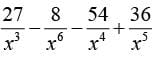will be,

Detailed Solution for Math Olympiad Test: Number System- 1 - Question 7

We have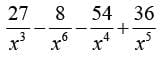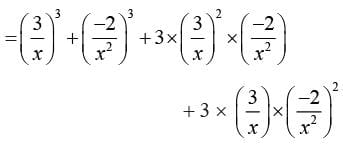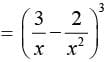∴ Cube root will be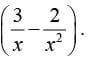Math Olympiad Test: Number System- 1 - Question 8

The volume of a cuboid is 3x2 - 27, its  possible dimensions  are

Detailed Solution for Math Olympiad Test: Number System- 1 - Question 8

Here 3x2 - 27 = 3(x2 - 9)
= 3[(x)2 - (3)2]
= 3(x + 3) (x - 3)

Math Olympiad Test: Number System- 1 - Question 9

If α + b + c ≠ 0, then the value of α3 + b3 + c3 - 3αbc will

Detailed Solution for Math Olympiad Test: Number System- 1 - Question 9

We have α3 + b3 + c3 - 3αbc
= (α + b + c)(α2 + b2 + c2 - αb - bc - cα)
If  (α + b + c) ≠ 0 then α3 + b3 + c3 - 3αbc will posses 0 value, if α2 + b2 + c2 - αb - bc - cα = 0, and this will only happen,
when α = b = c

Math Olympiad Test: Number System- 1 - Question 10

The value of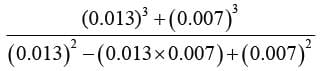Detailed Solution for Math Olympiad Test: Number System- 1 - Question 10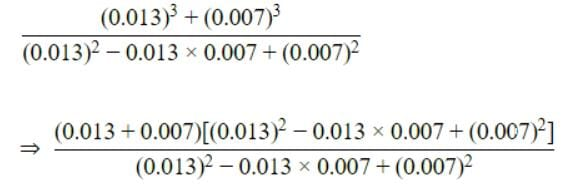[Since, (a)3 + (b)3 = (a + b) (a- ab + b2)]

⇒ (0.013 + 0.007)

⇒ 0.02

## Mathematics Olympiad for Class 9

1 videos|43 tests
 Use Code STAYHOME200 and get INR 200 additional OFF Use Coupon Code
Information about Math Olympiad Test: Number System- 1 Page
In this test you can find the Exam questions for Math Olympiad Test: Number System- 1 solved & explained in the simplest way possible. Besides giving Questions and answers for Math Olympiad Test: Number System- 1, EduRev gives you an ample number of Online tests for practice

## Mathematics Olympiad for Class 9

1 videos|43 tests